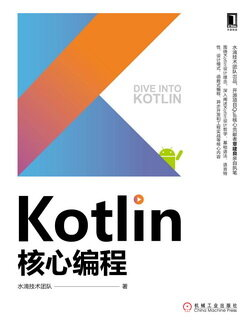# Kotlin 核心编程 (57)：面向对象 3.4.1(烦琐的 JavaBean)

public class Bird {    private double weight;    private int age;    private String color;     public void fly() {    }     public Bird(double weight, int age, String color) {        this.weight = weight;        this.age = age;        this.color = color;    }     public double getWeight() {        return weight;    }     public void setWeight(double weight) {        this.weight = weight;    }     public int getAge() {        return age;    }     public void setAge(int age) {        this.age = age;    }     public String getColor() {        return color;    }     public void setColor(String color) {        this.color = color;    }     @Override    public boolean equals(Object o) {        if (this == o)            return true;        if (!(o instanceof Bird))            return false;         Bird bird = (Bird) o;         if (Double.compare(bird.getWeight(), getWeight()) != 0)            return false;        if (getAge() != bird.getAge())            return false;        return getColor().equals(bird.getColor());    }     @Override    public int hashCode() {        int result;        long temp;        temp = Double.doubleToLongBits(getWeight());        result = (int) (temp ^ (temp > > > 32));        result = 31 * result + getAge();        result = 31 * result + getColor().hashCode();        return result;    }     @Override    public String toString() {        return "Bird{" +                "weight=" + weight +                ", age=" + age +                ", color='" + color + '\'' +                '}';    }}